# GSEB Solutions Class 10 Maths Chapter 15 Probability Ex 15.2

Gujarat Board GSEB Solutions Class 10 Maths Chapter 15 Probability Ex 15.2 Textbook Questions and Answers.

## Gujarat Board Textbook Solutions Class 10 Maths Chapter 15 Probability Ex 15.2

Question 1.
Two customers Shyam and Ekta are visiting a particular shop in the same week (Tuesday to Saturday). Each is equally likely to visit the shop on any day as on another day. What is the probability that both will visit the shop on:
(i) the same day?
(ii) consecutive days?
(iii) different days?
Solution:
Total possible ways of visiting shop by them = 5 x 5 = 25
(i) They can visit the shop on five weekdays Tuesday to Saturday.
Let A be the favorable outcomes of visiting shop by them on the same day = 5
Then, n(A) = 5
Therefore, P(A) = $$\frac {n( A)}{n(S)}$$ = $$\frac {5}{25}$$ = $$\frac {1}{5}$$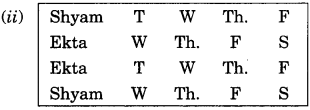Let A be the favorable outcomes to visiting shop on consecutive days.
Then, n(A) = 8
Therefore, P (A) = $$\frac {n( A)}{n(S)}$$ = $$\frac {5}{25}$$ = $$\frac {8}{25}$$

(iii) Let B be the favourable outcomes of visiting shop on the different days by them = 25 – 5 = 20 days i.e. n(B) = 20
Therefore, P(B) = $$\frac {n(B)}{n(S)}$$ = $$\frac {20}{25}$$ = $$\frac {4}{5}$$Question 2.
A die is numbered in such a way that its faces show the numbers 1, 2, 2, 3, 3, 6. It is thrown two times and the total score in two throws is noted. Complete the following table which gives a few values of the total score on the two throws: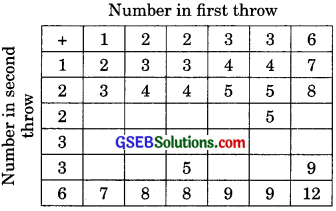What is the probability that the total score is (i) even? (ii) 6? (iii) at least 6?
Solution:
Number of possible outcomes = 36
i.e., n(S) = 36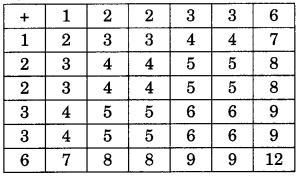1. Let A be the favorable outcomes that the total score is even.
Then n(A) = 18
Therefore, P(A) = $$\frac {n( A)}{n(S)}$$ = $$\frac {18}{36}$$ = $$\frac {1}{2}$$

2. Let B be favorable outcomes that the total score is 6.
Then, n( B) = 4
Therefore, P(B) = $$\frac {n(B)}{n(S)}$$ = $$\frac {4}{36}$$ = $$\frac {1}{9}$$

3. Let C be the favorable outcomes the total score is atleast 6.
Then, Therefore, P(C) = $$\frac {n(C)}{n(S)}$$ = $$\frac {15}{36}$$ = $$\frac {5}{12}$$Question 3.
A bag contains 5 red balls and some blue balls. If the probability of drawing a blue ball is double that of red ball, find the number of balls in the bag. (National Olympiad)
Solution:
Number of red balls in the bag = 5
Let number of blue balls in the bag = x
Total number of balls in the bag = x + 5
i.e., n(S) = x + 5
Let A be the favourable outcomes of getting red balls, then
n(A) = 5
Therefore, P(A) = $$\frac {n(A)}{n(S)}$$ = $$\frac {5}{x + 5}$$
Let B be the favourable outcomes of getting blue balls, then
n(B) = x
Therefore, P(B) = $$\frac {n(B)}{n(S)}$$ = $$\frac {x}{x + 5}$$
According to question,
P(B) = 2P(A)
⇒ $$\frac {x}{x + 5}$$ = 2 x $$\frac {x}{x + 5}$$
⇒ $$\frac {x}{x + 5}$$ = $$\frac {10}{x + 5}$$ ⇒ x = 10
Hence, the number of blue balls in the bag is 10.Question 4.
A box contains 12 balls out of which x are black.
1. If one ball is drawn at random, what is the probability that it will be a black ball?
2. If 6 more black balls are put in the box, the probability of drawing a black ball is now double of what it was before, find x.
Solution:
1. Given, a number of black balls = x and the total number of balls = 12.
∴ Probability of drawing a ball which is black,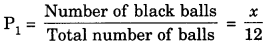2. If 6 more black balls are put in the box, then total number of black balls = x + 6 and total number of balls = 12 + 6 = 18.
∴ Probability of drawing a black ball,
P2 = $$\frac {x + 6}{18}$$
Now, according to the question,
P2 = 2P1
⇒ $$\frac {x + 6}{18}$$ = 2 x $$\frac {x}{12}$$
⇒ $$\frac {x + 6}{18}$$ = $$\frac {x}{6}$$
⇒ 6(x + 6) = 18x ⇒ x + 6 = 3x
⇒ 2x = 6 ⇒ x = 3Question 5.
Ajar contains 24 marbles, some are green, and others are blue. If a marble is drawn at random from the jar, the probability that it is green is $$\frac {2}{3}$$  Find the number of blue marbles in the jar.
Solution:
Let the number of green marbles = x
Then, the number of blue marbles = 24 -x
Total number of marbles = 24
Now, the probability of drawing a marble which is green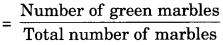= $$\frac {2}{3}$$ (Given)
⇒ $$\frac {x}{24}$$ = $$\frac {2}{3}$$
⇒ x = $$\frac {24 x 2}{3}$$
⇒ x = 16
∴ Number of green marbles = 18
Hence, number of blue marbles = 24 – 16 = 8.